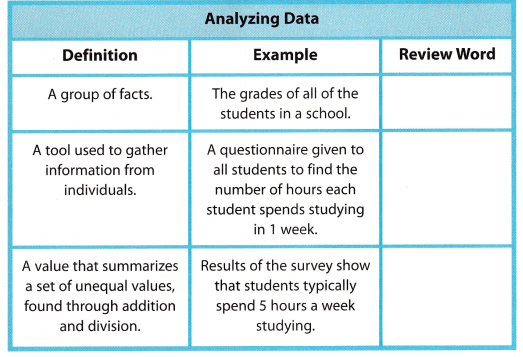Refer to our Texas Go Math Grade 8 Answer Key Pdf to score good marks in the exams. Test yourself by practicing the problems from Texas Go Math Grade 8 Module 15 Answer Key Sampling.

Find the percent.

Question 1.
20% of 50 ___________
20% = 20/100
$$\frac{20}{100}$$ × 50
2 × 5 = 10
So, 20% of 50 is 10

Question 2.
8.5% of 300 ____________
8.5% = 8.5/100
$$\frac{8.5}{100}$$ × 300
= 8.5 × 3
= 25.5
Therefore 8.5% of 300 is 25.5

Question 3.
175% of 16.8 ____________
175% = 175/100
$$\frac{175}{100}$$ × 16.8
= 1.75 × 16.8
= 29.4
Therefore 175% of 16.8 is 29.4

Solve for x.

Question 4.
$$\frac{x}{12}$$ = $$\frac{24}{36}$$ ____________
Given,
$$\frac{x}{12}$$ = $$\frac{24}{36}$$
x = $$\frac{24}{36}$$ × 12
x = 8

Question 5.
$$\frac{8}{x}$$ = $$\frac{16}{7}$$ ____________
Given,
$$\frac{8}{x}$$ = $$\frac{16}{7}$$
8 × $$\frac{7}{16}$$ = x
x = 7/2
x = 3.5

Question 6.
$$\frac{5}{6}$$ = $$\frac{x}{18}$$ ____________
Given,
$$\frac{5}{6}$$ = $$\frac{x}{18}$$
x = $$\frac{5}{6}$$ × 18
x = 5 × 3
x = 15

Question 7.
$$\frac{14}{15}$$ = $$\frac{x}{75}$$ ____________
Given,
$$\frac{14}{15}$$ = $$\frac{x}{75}$$
x = $$\frac{14}{15}$$ × 75
x = 14 × 5
x = 70

Find the mean of the data.

Question 8.
55, 44, 53, 62, 51: ____________
Given data 55, 44, 53, 62, 51
mean = sum of observations/number of observations
mean = (55 + 44 + 53 + 62 + 51)/5
mean = 265/5
mean = 53
Thus the mean of the data is 53.

Question 9.
3, 5, 3, 5, 2, 2, 5, 7: _____________
Given data 3, 5, 3, 5, 2, 2, 5, 7
mean = sum of observations/number of observations
mean = (3 + 5 + 3 + 5 + 2 + 2 + 5 + 7)/8
mean = 32/8
mean = 4
Thus the mean of the data is 4.

Visualize Vocabulary

Use the ✓ words to complete the right column of the chart.Understand Vocabulary

Complete the sentences using the preview words.

Question 1.
The entire group of objects, individuals, or events is the ____________.
Answer: The entire group of objects, individuals, or events is the population.

Question 2.
A sample in which every person, object, or event has an equal chance at being selected is a ___________.
Answer: A sample in which every person, object, or event has an equal chance at being selected is a simple random sample.